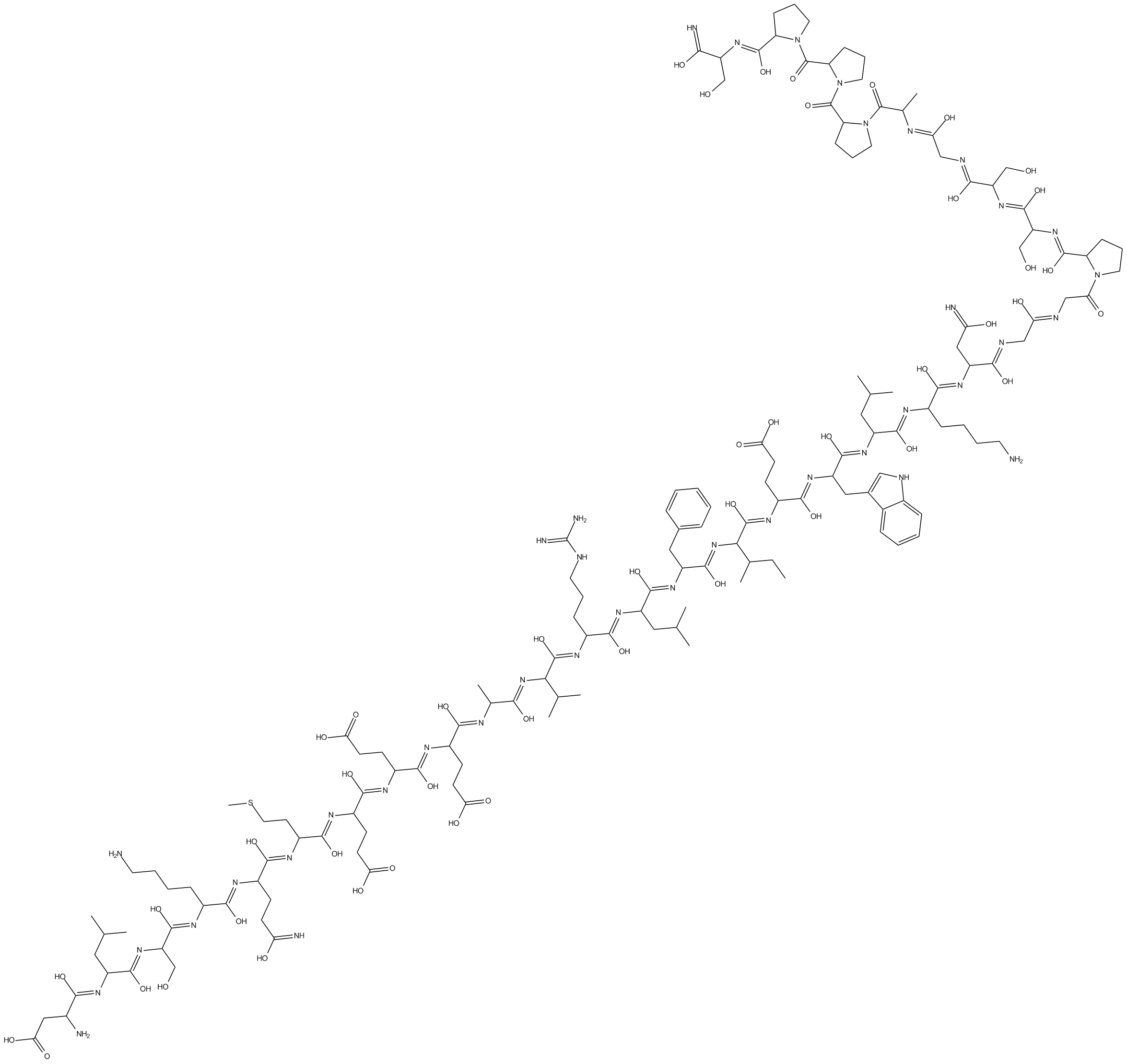• 菜单
• Setting

# Exendin-3 (9-39) amide

Catalog No.
B6943
GLP-1受体拮抗剂1mg
￥ 2,700.00

## 文献引用

1. Jie Wu, Pingfan Guo, et al. "Glucagon-like peptide-1 affects human umbilical vein endothelial cells in high glucose by the PI3K/Akt/eNOS signaling pathway." Turk J Biochem. 2017 09 11.

## Chemical Properties

 Physical Appearance A solid Storage Desiccate at -20°C M.Wt 3369.79 Cas No. 133514-43-9 Formula C149H234N40O47S Solubility ≥168.5mg/mL in DMSO SDF Download SDF Canonical SMILES CCC(C(/N=C(O)/C(/N=C(O)/C(/N=C(O)/C(/N=C(O)/C(/N=C(O)/C(/N=C(O)/C(/N=C(O)/C(/N=C(O)/C(/N=C(O)/C(/N=C(O)/C(/N=C(O)/C(/N=C(O)/C(/N=C(O)/C(/N=C(O)/C(N)CC(O)=O)CC(C)C)CO)CCCCN)CCC(O)=N)CCSC)CCC(O)=O)CCC(O)=O)CCC(O)=O)C)C(C)C)CCCNC(N)=N)CC(C)C)CC1=CC=CC=C1)/C( 运输条件 试用装：蓝冰运输。 其他可选规格：常温运输或根据您的要求用蓝冰运输。 一般建议 为了使其更好的溶解，请用37℃加热试管并在超声波水浴中震动片刻。不同厂家不同批次产品溶解度各有差异，仅做参考。若实验所需浓度过大至产品溶解极限，请添加助溶剂助溶或自行调整浓度。

## 质量控制

### 化学结构